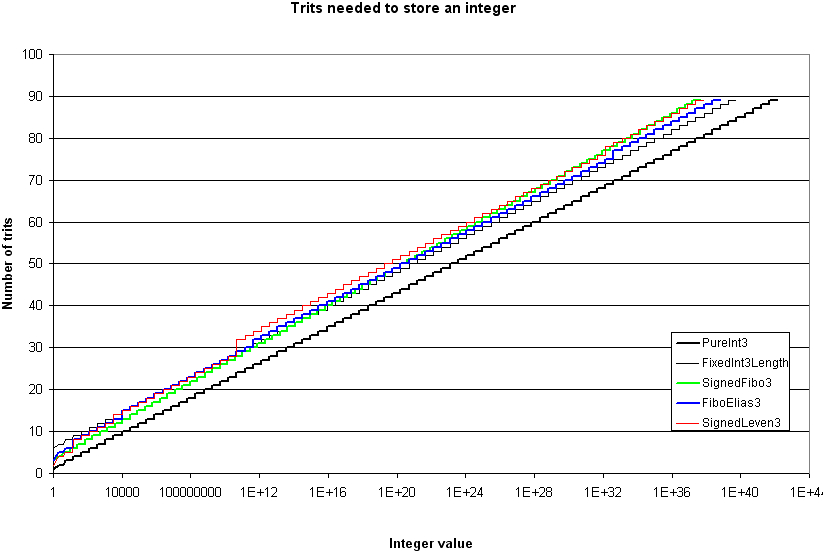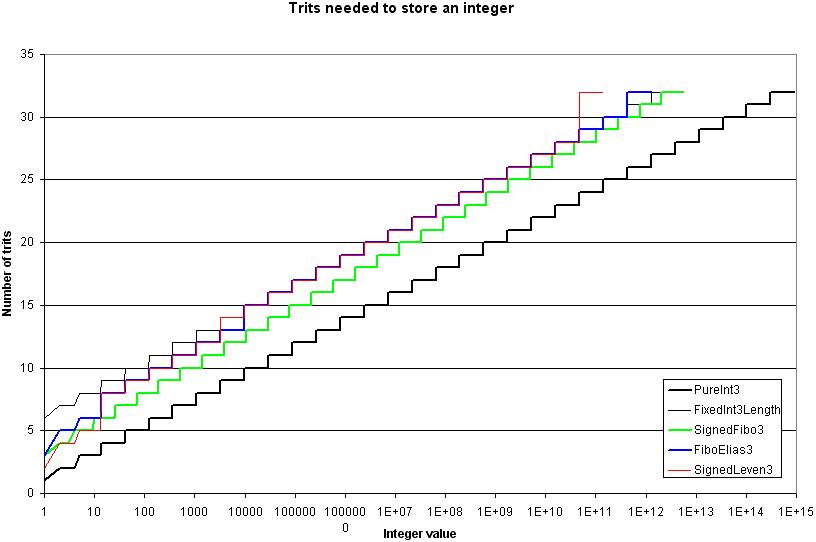Users >> Pisto-UniversalCodes
Pisto-Universal Codes

The C++ codes listed here are protected by GPL v3. The algorithms behind these codes should remain free of any patent.

## Universal Codes to Compress Data

Please, take a look at this topic on Wikipedia.org, and look at the graphs on the right of that topic's page.

Once it is done, the current page on 3niti.org will present you three different universal codes adapted for balanced ternary integers: Fibonacci, Hybrid Fibonacci/Elias (my own creation) and Levenstein.

These universal codes could be used to compress an array of integers that are generally very close to 0. In page Pisto-DataTypes, I have introduced the concept of encoding two integer components in a single integer. This could be useful for addresses (ID+Address) and for compacted/compressed float (`cfloat`: exponent+mantissa).

With the results shown in the current page, I am not able to suggest one preferable universal code for 90-trits integers. I will let the choice open.

### Fibonacci Code with Trits - SignedFibo3

First of all, the name "Fibonacci" may not be appropriate for the coding method with trits, since the current method no longer uses numbers of the Fibonacci sequence. Maybe we should call it "Zeckendorf coding". In fact, the Zeckendorf's theorem is the basis of the Fibonacci coding. On the other hand, the sequence that is used in the current method (A028859) has been found by many other researchers (including myself). So, maybe it is simplest calling the current method "Fibonacci" or SignedFibo3.

The main characteristic of this coding method is that we have a criterion that tells us where the code stops. In the original method for bits, the last two bits of an encoded integer are "11". So, we can decode the integer until we reach two consecutive bits "1". All bits except the last one are multiply by their corresponding Fibonacci number, and all products are added together. All this works because of the Zeckendorf's theorem. Fortunately, there is an equivalent for ternary integers.

For ternary integers, the criterion that ends the code is "`PP`". As in the original method for bits, the method only encodes positive integers. In order to encode negative numbers, and value 0, we must prepend a trit which tells us the sign.

Before going any further, let me introduce you the chosen sequence (A028859):

``` 1 3 8 22 60 164 ...

f(0) = 1
f(1) = 3
f(n) = 2 * (f(n-1) + f(n-2))
```

Here is an example with integer 40:

Sequence Trits Products Sum 40 1 3 8 22 60 `N` `P` `O` `N` `P` -1 3 0 -22 60 `P NPONPP`

In the previous table, the first "`P`" stands for the sign (positive), and the last "P" is the stopping criterion. So, to encode 40, we need 7 trits. Integer -40 would have been encoded as "`N NPONPP`" instead.

Here is a table containing the maximum value for each number of trits (the sign counts in the number of trits):

Nb TritsSignFibo3 CodeValue
1`O`` `0
3`P``PP`1
4`P``OPP`3
5`P``POPP`9
6`P``OPOPP`25
7`P``POPOPP`69
8`P``OPOPOPP`189
9`P``POPOPOPP`517
10`P``OPOPOPOPP`1413
11`P``POPOPOPOPP`3861
12`P``OPOPOPOPOPP`10549
13`P``POPOPOPOPOPP`28821
14`P``OPOPOPOPOPOPP`78741
15`P``POPOPOPOPOPOPP`215125
16`P``OPOPOPOPOPOPOPP`587733
............
47`P``...POPOPOPOPOPP`1.99679e+019
48`P``...OPOPOPOPOPOPP`5.45533e+019
............
89`P``...POPOPOPOPOPOPP`4.29399e+037

Please note that code "`P NNNNN...NNNPP`" is allowed, since the stopping criterion is only "`PP`". It would have been possible to use two stopping criteria (`PP` and `NN`) instead of using an additional trit for the sign, but this method would have generated longer codes. So, we are better keeping the sign apart, since it gives us the opportunity to encode value "0" with only one trit.

The way it should work for cfloat in CPU's multimedia registers is described in the following table:

cfloat with SignedFibo3-coded exponent
SignExponent (absolute value)Mantissa
`O` `LST ................ MST`
`P` or `N`(`P`?(`O` or `N`))*`PP``LST ................ MST`
Min. 1 trit, max. 89 tritsMin. 1 trit, max. 89 trits
Min. 2 trits, max. 90 trits

In practice, we need at least 4 trits for the whole `cfloat`, because we need to support all the following cases: +Inf, -Inf, NaN, -1, 0, 1. If not, the 2-trits `cfloat` would only support -1, 0, 1 and NaN (because the encoded exponent would be corrupted if it is not `O`).

With at least 4 trits, the NaN case is defined by a `O` at the most significant trit of the mantissa, but the encoded floating point value is not 0 (which is all made of "`O`"s). For example: `PPPO` is NaN because the most significant trit of the mantissa is "`O`", but the encoded value is not 0. Finally, if the Fibo3 code is corrupted (the sign is `P` or `N`, but the whole `cfloat` contains no "`PP`"), then this is NaN.

The + or - Infinity is defined by a sign `P` or `N` and a valid Fibo3 code that leaves no trit for the mantissa. Then, the sign of the exponent becomes the sign of Infinitiy. For example with 4 trits: `NOPP` is -Inf, and `POPP` is +Inf.

### Hybrid Fibonacci/Elias Code with Trits - FiboElias3

There are three main Elias codes: Elias gamma code, Elias delta code and Elias omega code. All these methods have the following structure:

• A code (universal or not) to encode the length of the integer;
• The integer without its most significant bit.

In the hybrid method I am presenting here, I have used the (unsigned) Fibo3 code (previous section) to encode the length of the integer. The sign is given by the integer itself, so all trits are preserved. Finally, everything is encoded in little endian.

Here is a table containing the maximum value for each number of trits:

Nb TritsLengthIntegerValue
3`PP``O`0
3`PP``P`1
5`NPP``PP`4
6`OPP``PPP`13
8`NNPP``PPPP`40
9`ONPP``PPPPP`121
10`PNPP``PPPPPP`364
11`NOPP``PPPPPPP`1093
12`OOPP``PPPPPPPP`3280
13`POPP``PPPPPPPPP`9841
15`NNNPP``PPPPPPPPPP`29524
16`ONNPP``PPPPPPPPPPP`88573
17`PNNPP``PPPPPPPPPPPP`265720
18`NONPP``PPPPPPPPPPPPP`797161
............
47`OPONPP``...PPPPPPPPPPP`1.82365e+019
48`NNPNPP``...PPPPPPPPPPPP`5.47095e+019
............
89`OOONNPP``...PPPPPPPPPPPPP`6.6514e+038

The way it should work for cfloat in CPU's multimedia registers is described in the following table:

cfloat with FiboElias3-coded exponent
LengthSigned ExponentMantissa
(`P`?(`O` or `N`))*`PP``LST ......... MST``LST ................ MST`
Min. 3 trits, max. 89 tritsMin. 1 trit, max. 87 trits
Min. 4 trits, max. 90 trits

Here are the special cases for the smallest cfloat with FiboElias3-coded exponent:

 -1: `PP O N` 0: `PP O O` 1: `PP O P` -Inf: `PP P N` Inf: `PP P P` NaN: `PP (P|N) O` or corrupted FiboElias3 code

### Levenstein Code with Trits - SignedLeven3

As the Elias Omega code, the Levenstein method is a technique that recursively encodes the length of the last prepended integer in a code. In other words, you write down the integer you want to encode. Then, you write down the number of bits or trits that have just been written down, minus 1. And so on, until you obtain a one or two bits or trits integer. Finally, you use 0 or O to finalize the code.

In the case of the Levenstein code, the value 0 is encoded as 0. Furthermore, all most significant bits or trits of all written integers are placed together at the beginning of the code. This let us use the little endian encoding. Here is an example with bits in little endian:

``` 10 in base 10 is "1010" in base 2 or "0101" in little endian:

0101 -> 4 bits, encode 4-1=3
11 -> 2 bits, encode 2-1=1
1 -> 1 bit, encode 1-1=0
0 -> end of process

0 1 11 0101 -> Take all most significant bits

0 1  1    1 -> reverse the order -> 1110
_ _ 1_ 010_

Finale code: 1110 1 010
```

What happens when using trits instead of bits? The original integer is written as it is. Then, the number of written trits, minus 1, is the next number to write down. But, before we write it down, we may discard the most significant trit `P` only if the second most significant trit is a `N`. Finally, when we reach the trit `O` (end of process), all remaining most significant trits are placed together in the header of the code. Here is an example:

``` 365 in base 10 is NNNNNNP in balanced ternary notation:

Even if the original integer satisfies the (N|O|P)*NP pattern, keep all its trits.
This is mandatory since we want to encode (and being able to decode) signed integers.
NNNNNNP -> 7 trits, encode 7-1=6
ONP -> Pattern (N|O|P)*NP is present, so get rid of the last P
ON -> 2 trits, encode 2-1=1
P -> Pattern (N|O|P)*NP is absent
P -> 1 trit, encode 1-1=0
O -> Pattern (N|O|P)*NP is absent
O -> End of process

O P ON NNNNNNP -> Take all most significant trits

O P  N       P -> reverse the order -> PNPO (the header)
_ _ O_ NNNNNN_ -> The body

Finale code: PNPO O NNNNNN
```

Here is a table containing the maximum value for each number of trits:

1`O`` `0
2`PO`` `1
4`PPO``P`4
5`PNO``PP`13
8`PPPO``O PPP`40
9`PPPO``P PPPP`121
10`PNPO``N PPPPP`364
11`PNPO``O PPPPPP`1093
12`PNPO``P PPPPPPP`3280
14`PPNO``NO PPPPPPPP`9841
15`PPNO``OO PPPPPPPPP`29524
16`PPNO``PO PPPPPPPPPP`88573
17`PPNO``NP PPPPPPPPPPP`265720
18`PPNO``OP PPPPPPPPPPPP`797161
............
89`PPPPO``P PNOO PPP...PPP`7.39044e+037

We may observe that the first trit of the header is the sign of the encoded integer.

The way it should work for cfloat in CPU's multimedia registers is described in the following table:

cfloat with SignedLeven3-coded exponent
(`P` or `O` or `N`)*`O`...`LST ................ MST`
Min. 1 trits, max. 89 tritsMin. 1 trit, max. 89 trits
Min. 2 trits, max. 90 trits

To support -Inf, -1, 0, 1, +Inf and NaN, we need at least 3 trits for the whole cfloat:

 -1: `O ON` 0: `O OO` 1: `O OP` -Inf: `PO N` Inf: `PO P` NaN: `(P|N)O O` or corrupted SignedLeven3 code

### Comparison

The three previous coding methods for integer numbers are now compared together with two other types of codes which are not universal: `PureInt3`, which is the integer written normally, and `FixedInt3Length`, which is like a Elias code (length+integer) with the length of the integer that is always encoded with 5 trits. I have chosen 5 trits because, in our application, the maximum number of trits is less than 121 (PPPPP).

The comparison has been done with these C++ files.

The next graph shows, for each coding method, the number of trits needed for each value greater than 0 (because of the semi-log graph).The next graph stops at 32 trits, so it looks like a zoom on smaller values of the previous graph:So! Which coding method is the best?

• SignedLeven3 is the best from 2 to 5 trits.
• Ultimately, for very very large integers (not shown in these graphs), the SignedLeven3 coding method is the most compact one.
• With 89 trits, the FiboElias3 method encodes the greatest integers, but because this last method is based on Fibo3, which is not asymptotically optimal, FiboElias3 will ultimately use more trits than SignedLeven3 to encode very very large integers.
• SigneFibo3 is the best choice from 6 to 47 trits. We can see this in previous tables of results and in the first graph.
• For large integers (48 to 89 trits), the best coding method is the hybrid FiboElias3 method.

While none of the previous method is optimal for all cases, it is practically possible to choose the best of them according to the length of a `cfloat`:

• `cfloat<3>` to `cfloat<6>`: `SignedLeven3` for the exponent and at least one trit for the mantissa;
• `cfloat<7>` to `cfloat<48>`: `SignedFibo3` for the exponent and at least one trit for the mantissa;
• `cfloat<49>` to `cfloat<90>`: `FiboElias3` for the exponent and at least one trit for the mantissa;

## Conclusion

While all this theory is very interesting (my personnal opinion), I did not talk about how it should be implemented in processors. In fact, which method is the easiest and the fastest one to compute? Another, more important, question: is all this really useful for scientists and programmers? I will leave these questions open.